# 从图(Graph)到图卷积(Graph Convolution)：漫谈图神经网络模型 (三)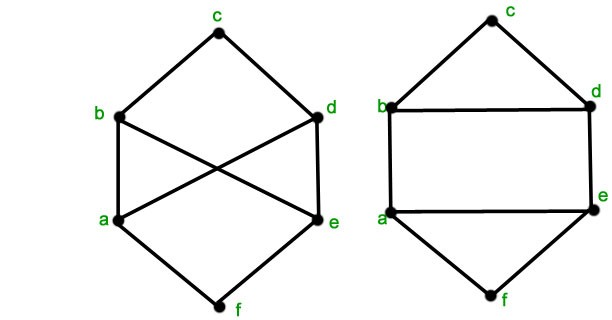## 基于统计的方法(Statistics Category)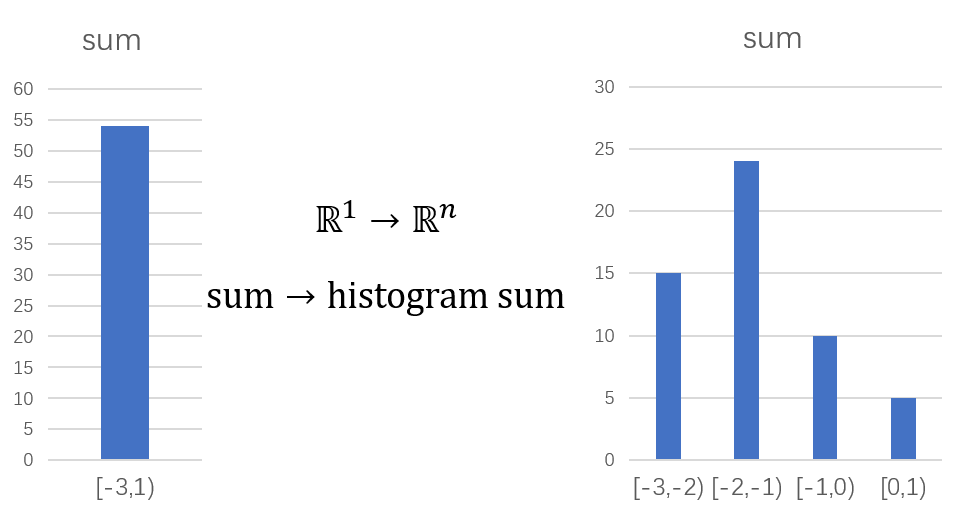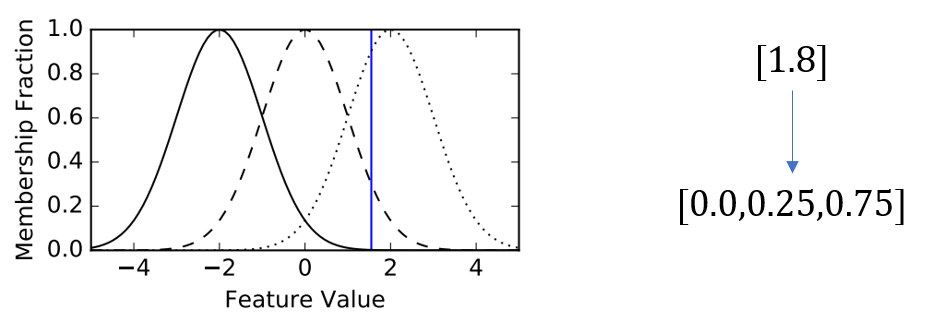## 基于学习的方法(Learning Category)

### 采样加全连接(Sample And FC)

$\textbf{h}_𝐺=FC(\textbf{H}^𝐿)$

### 全局结点(Global Node)

1. 很多情况下我们很难找到一个合适的根结点（笔者觉得图的根结点一般都是根据各个领域的领域知识来确定的，比如我们在第一篇博客中讲到的化合物分类问题）
2. 如果直接用基于统计的方法对各个结点一视同仁，无法区别对待（比如某些重要的结点信息更多，就应该表达得更多）。

### 可微池化(Differentiable Pooling)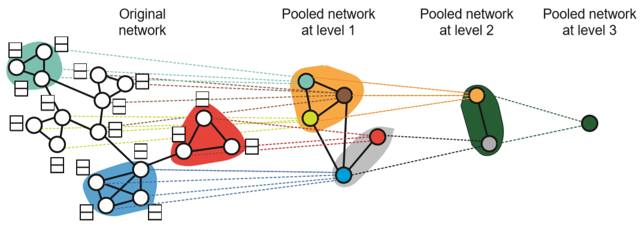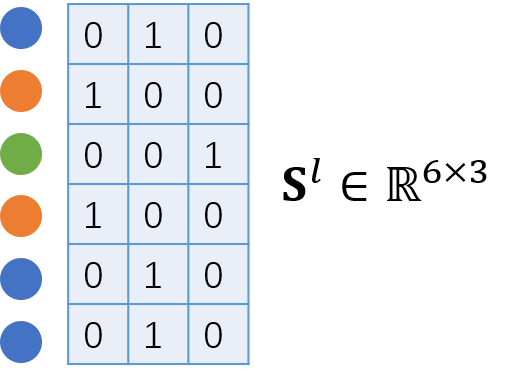$$\textbf{A}^l$$表示第$$l$$层子图结点的邻接关系，$$\textbf{A}^0$$即是图的邻接矩阵，$$N_l$$表示第$$l$$层子图结点的个数，$$\textbf{H}^l$$表示第$$l$$层子图各个结点表示堆叠而成的隐状态矩阵，DiffPool通过如下公式得到新子图中各个结点的表示：

$\textbf{S}^l=SC(\textbf{A}^l,\textbf{H}^l), 其中 \textbf{S}^l{\in}\mathbb{R}^{(N_l×N_{l+1})}$

$\tilde{\textbf{H}}^l = NR (\textbf{A}^l,\textbf{H}^l)$

$\textbf{H}^{l+1}=(\textbf{S}^l)^T\tilde{\textbf{H}}^l$

$\textbf{A}^{l+1}=(\textbf{S}^l)^T{\textbf{A}^l}\textbf{S}^l$

## 参考文献

. Molecular graph convolutions moving beyond fingerprints, https://arxiv.org/abs/1603.00856

. Hierarchical Graph Representation Learning with Differentiable Pooling, https://arxiv.org/abs/1806.08804

posted @ 2019-09-07 17:43  SivilTaram  阅读(28096)  评论(22编辑  收藏  举报